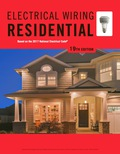# For the range discussed in problem 11: a. What size 60°C ungrounded conductors are required? ______________ b. What size 60°C neutral conductor is required? ______________ c. What size 75°C ungrounded conductors are required? The terminal block and lugs on the range, the branch-circuit breaker, and the panelboard are marked as suitable for 75°C wire. ______________ d. What size 75°C neutral conductor is required? Terminations are the same as in part (c). ______________### EBK ELECTRICAL WIRING RESIDENTIAL

19th Edition
Simmons
Publisher: CENGAGE LEARNING - CONSIGNMENT
ISBN: 9781337516549

#### Solutions

Chapter
Section### EBK ELECTRICAL WIRING RESIDENTIAL

19th Edition
Simmons
Publisher: CENGAGE LEARNING - CONSIGNMENT
ISBN: 9781337516549
Chapter 20.2, Problem 12R
Textbook Problem
4 views

## For the range discussed in problem 11: a. What size 60°C ungrounded conductors are required? ______________ b. What size 60°C neutral conductor is required? ______________ c. What size 75°C ungrounded conductors are required? The terminal block and lugs on the range, the branch-circuit breaker, and the panelboard are marked as suitable for 75°C wire. ______________ d. What size 75°C neutral conductor is required? Terminations are the same as in part (c). ______________

a.

To determine

State the required size of 60°C ungrounded conductors.

### Explanation of Solution

Given data:

Refer problem 11 in the textbook.

Discussion:

The power rating of a double-oven electric range is 18kW. Refer table 220.55 in the textbook, the power rating of a double-oven (18kW) exceeds the maximum demand in column C (12kW) by (6kW). It is given that the maximum demand is 8kW for one number of appliance.

From table 220.55 note 1, each additional kilowatt of rating from the maximum demand in column C shall be increased by 5%. Therefore, the total percentage of increase of demand is (6×5%=30%). Hence, the amount of increase in demand is (8kW×0.3=2

b.

To determine

State the required size of 60°C neutral conductor.

c.

To determine

State the required size of 75°C ungrounded conductor.

d.

To determine

State the required size of 75°C neutral conductor.

### Still sussing out bartleby?

Check out a sample textbook solution.

See a sample solution

#### The Solution to Your Study Problems

Bartleby provides explanations to thousands of textbook problems written by our experts, many with advanced degrees!

Get Started

Find more solutions based on key concepts
What is cloud computing? What are some pros and cons of cloud computing?

Principles of Information Systems (MindTap Course List)

Write the script for a one-minute explanation of basic project management concepts.

Systems Analysis and Design (Shelly Cashman Series) (MindTap Course List)

Solve the following set of equations using the Gaussian method. {x1x2x3}={61514}

Engineering Fundamentals: An Introduction to Engineering (MindTap Course List)

In a typical database system, each department or area within an organization has its own set of files. (504)

Enhanced Discovering Computers 2017 (Shelly Cashman Series) (MindTap Course List)

If your motherboard supports ECC DDR3 memory, can you substitute non-ECC DDR3 memory?

A+ Guide to Hardware (Standalone Book) (MindTap Course List)

What is the EPEAT? How is it used?

Principles of Information Systems (MindTap Course List)